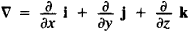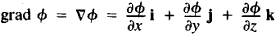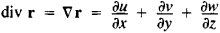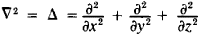# Hamiltonian Operator

## Hamiltonian operator

[‚ham·əl′tō·nē·ən ¦äp·ə‚rād·ər]
(quantum mechanics)
McGraw-Hill Dictionary of Scientific & Technical Terms, 6E, Copyright © 2003 by The McGraw-Hill Companies, Inc.
The following article is from The Great Soviet Encyclopedia (1979). It might be outdated or ideologically biased.

## Hamiltonian Operator

(also del, or ▽-operator), a differential operator of the formwhere i, j, and k are coordinate unit vectors. It was introduced by Sir W. R. Hamilton in 1853. If the Hamiltonian operator is applied to a scalar function φ (x, y, z) and ▽φ is understood to be the product of a vector and a scalar, the gradient of the function is produced:If the operator is applied to a vector function r(x, y, z), when ▽ r is understood to be the scalar product of vectors, the divergence of the vector r is produced:(u, v, and w are the coordinates of the vector r). The scalar product of the Hamiltonian operator and itself gives the Laplacian operator:References in periodicals archive ?
The inhomogeneous Schrodinger equation, where H = [H.sub.0] + V is the Hamiltonian operator, can be expressed as
Moreover, in the lattice soliton theory, if we can seek out a Hamiltonian operator J and a series of conserved functionals [[??].sup.(m).sub.n] (m [greater than or equal to] 1) so that (4) maybe represented as the following forms
The expression exactly solvable means the eigenvalues and the eigenfunctions of the Hamiltonian operator of the physical system can be derived analytically in closed form.
The quantum path integral formulation [36, 39-42] is based on the partition function of the quantum statistical canonical ensemble which is defined as the trace of the exponential of the Hamiltonian operator:
More precisely, the defect sets [mathematical expression not reproducible] are actually described, and, in addition, these results are applied to a Hamiltonian operator matrix.
is said to be a partial Hamiltonian operator corresponding to a current value Hamiltonian H(t, [q.sup.i],[p.sub.i],[c.sub.i]) which satisfies (2.2), if there exists a function B(t,[q.sup.i],[p.sub.i]) such that
When a quantum well is in the presence of a monochromatic laser beam--linearly polarized in the axial direction (z axis)--we can study the electronic states of the confinement potential and the electromagnetic field, including the term representing the external field in the kinetic part of the Hamiltonian operator. This semiclassical operation is a combination of the quantum description of a particle motion and the classical description of the radiation field.
However, payoff has to be real number due to the limitation of Hamiltonian operator (explained in Section 2.4).
The second step is to introduce the related Hamiltonian operator:
Keywords: Self adjoiness, Hamiltonian operator, potential, Hilbert space, Norm, Laplace Operator.

Site: Follow: Share:
Open / Close